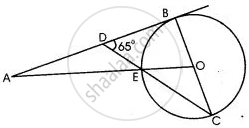Share

# In the Adjoining Figure, O is the Centre of the Circle and Ab is a Tangent to It at Point B. ∠Bdc = 65° Find ∠Bao. - Mathematics

Course

#### Question

In the adjoining figure, O is the centre of the circle and AB is a tangent to it at point B. ∠BDC = 65° Find ∠BAO.#### Solution 1

AB is a straight line.
∴ ∠ADE +  ∠BDE = 180°
⇒ ∠ADE  + 65° = 180°
⇒ ∠ADE = 115°          ………..(i)
AB i.e. DB is tangent to the circle at point B and BC is the diameter.

∴∠DB = 90°
In ΔBDC,
∠DBC +  ∠BDC + ∠DCB = 180°
⇒ 90° +  65° +  ∠DCB  = 180°
⇒ ∠ DCB = 25°
Now, OE = OC (radii of the same circle)
∴ ∠DCB or ∠OCE =  ∠OEC = 25°
Also,
∠OEC = ∠DEC =  25°

(vertically opposite angles)
∠ADE + ∠DEA + ∠DAE = 180°
From (i) and (ii)
115° + 25°  + ∠ DAE  = 180°
⇒ ∠DAE or ∠BAO  = 180° - 140° =40°
∴∠BAO = 40°

#### Solution 2

As AB is a tangent to the circle at B and OB is radius, OB + AB ⇒ ∠ CBD = 90°.

In ΔBCD,
∠ BCD + ∠ CBD + ∠ BDC = 180°
∠ BCD + 90° + 65° = 180°
∠ BCD + 155° = 180°
∠ BCD = 180° - 155°
∠ BCD = 25°
∠ BOE = 2∠ BCE      ....(angle at centre = double the angle at the remaining part of circle)
∠ BOE = 2 x 25°
∠ BOE = 50°
∠ BOA = 50°

In ΔBOA,
∠ BAO + ∠ ABO + ∠ BOA = 180°
∠ BAO + 90° + 50° = 180°
∠ BAO + 140° = 180°
∠ BAO = 180° - 140°
∠ BAO = 40°

Is there an error in this question or solution?